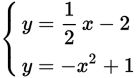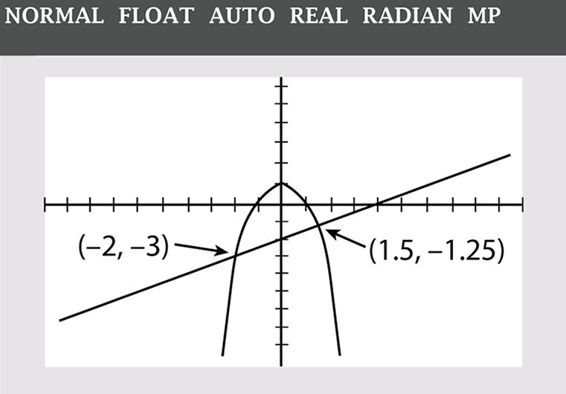# SAT Math Multiple Choice Question 499: Answer and Explanation

### Test Information

Question: 499

4.If (a, b) is a solution to the system of equations above, which of the following could be the value of b?

• A. -3
• B. -2
• C. 1
• D. 2

Explanation:

A

Difficulty: Medium